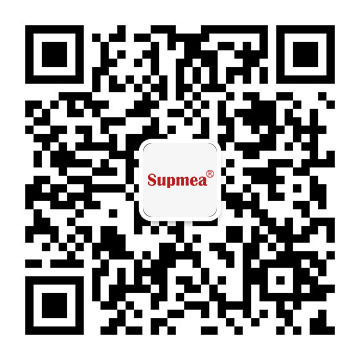# Introduction of Conductivity meter

What principle knowledge should be mastered during the use of the conductivity meter? First, in order to avoid electrode polarization, the meter generates a highly stable sine wave signal and applies it to the electrode. The current flowing through the electrode is proportional to the conductivity of the measured solution. After the meter converts the current from a high-impedance operational amplifier into a voltage signal, After program-controlled signal amplification, phase-sensitive detection and filtering, the potential signal reflecting the conductivity is obtained; the microprocessor switches through the switch to alternately sample the temperature signal and the conductivity signal. After calculation and temperature compensation, the measured solution is obtained at 25°C. The conductivity value at the time and the temperature value at the time.

The electric field that causes the ions to move in the measured solution is generated by the two electrodes that are in direct contact with the solution. The pair of measuring electrodes must be made of chemical resistant materials. In practice, materials such as titanium are often used. The measuring electrode composed of two electrodes is called the Kohlrausch electrode.

The measurement of conductivity needs to clarify two aspects. One is the conductivity of the solution, and the other is the geometric relationship of 1/A in the solution. The conductivity can be obtained by measuring current and voltage. This measurement principle is applied in today's direct display measuring instruments.

And K=L/A

A——The effective plate of the measuring electrode
L——The distance between the two plates

The value of this is called the cell constant. In the presence of a uniform electric field between the electrodes, the electrode constant can be calculated by geometric dimensions. When two square plates with an area of 1cm2 are separated by 1cm to form an electrode, the constant of this electrode is K=1cm-1. If the conductivity value G=1000μS measured with this pair of electrodes, then the conductivity of the tested solution K=1000μS/cm.

Under normal circumstances, the electrode often forms a partial non-uniform electric field. At this time, the cell constant must be determined with a standard solution. Standard solutions generally use KCl solution. This is because the conductivity of KCl is very stable and accurate under different temperatures and concentrations. The conductivity of 0.1mol/l KCl solution at 25°C is 12.88mS/CM.

The so-called non-uniform electric field (also called stray field, leakage field) has no constant, but is related to the type and concentration of ions. Therefore, a pure stray field electrode is the worst electrode, and it cannot meet the needs of a wide measurement range through one calibration.

2. What is the application field of the conductivity meter?

Applicable fields: It can be widely used in continuous monitoring of conductivity values ​​in solutions such as thermal power, chemical fertilizers, metallurgy, environmental protection, pharmaceuticals, biochemicals, food and tap water.

3.What is the cell constant of the conductivity meter?

"According to the formula K=S/G, the cell constant K can be obtained by measuring the conductance G of the conductivity electrode in a certain concentration of KCL solution. At this time, the conductivity S of the KCL solution is known.

The electrode constant of the conductivity sensor accurately describes the geometric properties of the two electrodes of the sensor. It is the ratio of the length of the sample in the critical area between the 2 electrodes. It directly affects the sensitivity and accuracy of the measurement. The measurement of samples with low conductivity requires low cell constants. The measurement of samples with high conductivity requires high cell constants. The measuring instrument must know the cell constant of the connected conductivity sensor and adjust the reading specifications accordingly.

4. What are the cell constants of the conductivity meter?

The two-electrode conductivity electrode is currently the most widely used type of conductivity electrode in China. The structure of the experimental two-electrode conductivity electrode is to sinter two platinum sheets on two parallel glass sheets or the inner wall of a round glass tube to adjust the platinum sheet Area and distance can be made into conductivity electrodes with different constant values. Usually there are K=1, K=5, K=10 and other types.

The principle of the conductivity meter is very important. When choosing a product, you must also choose a good manufacturer.

back
##### Inquiry
Phone
+86 15868103947
WeChatTOP